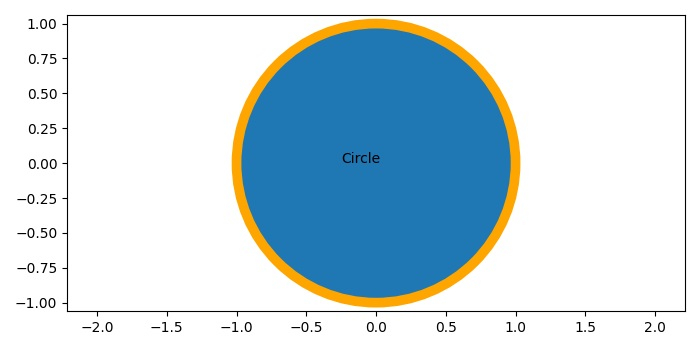# Plot a circle with an edgecolor in Matplotlib

To plot a circle with an edgecolor in matplotlib, we can take the following Steps −

• Create a new figure or activate an existing figure using figure() method.

• Add a subplot method to the current axis.

• Create a circle instance using Circle() class with an edgecolor and linewidth of the edge.

• Add a circle path on the plot.

• To place the text in the circle, we can use text() method.

• Scale the X and Y axes using xlim() and ylim() methods.

• To display the figure, use show() method.

## Example

import matplotlib
from matplotlib import pyplot as plt, patches
plt.rcParams["figure.figsize"] = [7.00, 3.50]
plt.rcParams["figure.autolayout"] = True
fig = plt.figure()
plt.show()